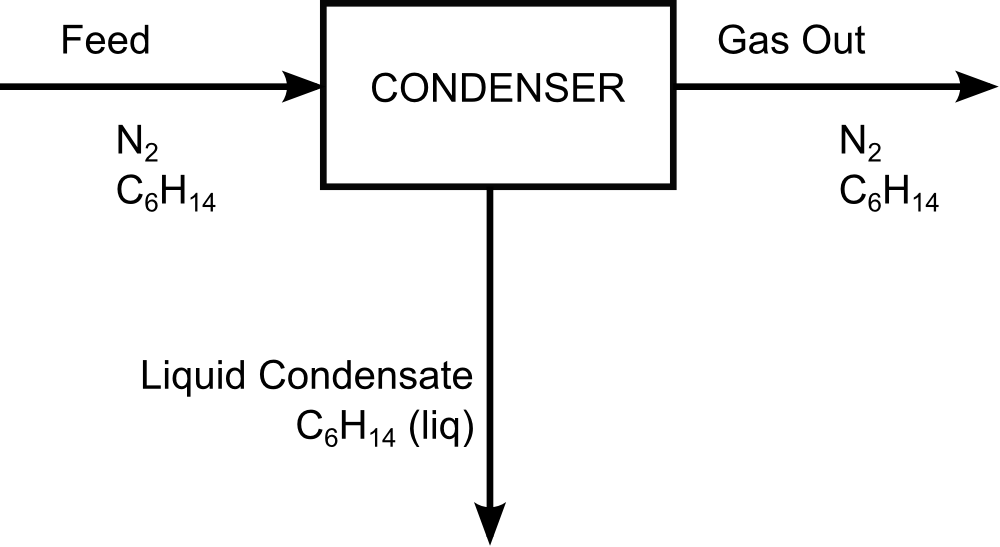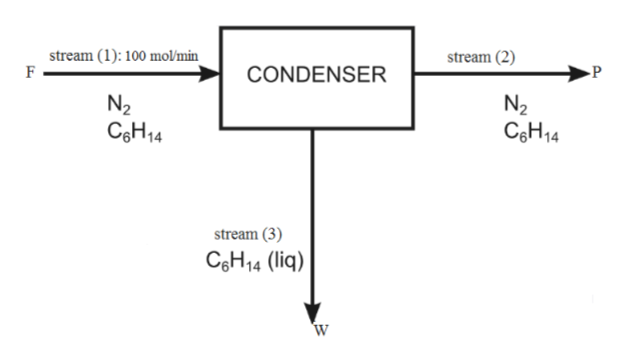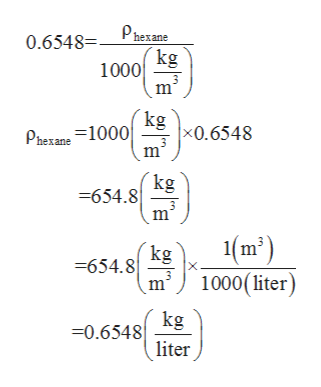# Gas OutFeedCONDENSERN2CeH14N2CeH14Liquid CondensateCgH14 (liq)

Question
10 views

gas stream contains 15.0 mole% hexane and the remainder nitrogen. The stream flows to a condenser, where its temperature is reduced and some of the hexane is liquefied. The hexane mole percent in the gas stream leaving the condenser is 6.00. Liquid hexane condensate is recovered at a rate of 1.00 L/min. You may take the specific gravity of liquid hexane to be 0.6548.

What is the flow rate of the gas stream leaving the condenser in mol/min?  mol/min

What percentage of hexane entering the condenser is removed as a liquid? %help_outlineImage TranscriptioncloseGas Out Feed CONDENSER N2 CeH14 N2 CeH14 Liquid Condensate CgH14 (liq) fullscreen
check_circle

Step 1

Condenser is a device which is used to condense the vapors  into liquid state by reducing the temperature  (transferring heat).

Let molar flow rate of stream 1( feed stream ) = 100mol/min

Mole percent  of hexane = 15 %

Therefore , mole percent of nitrogen becomes = 85 %help_outlineImage Transcriptionclosestream (2) stream (1): 100 mol/min F P CONDENSER N2 N2 C&H14 C H14 stream (3) C&H14 (liq) W fullscreen
Step 2

Mole percent of hexane in stream (2) = 6%

Therefore, mole percent of nitrogen in stream (2) = 94%

In stream (3) only hexane is present and volumetric flow rate of hexane is given 1 liter/min

Specific gravity of hexane is given 0.6548

Specific gravity = density of substance /density of water

Density of water = 1000 kg/m3

1 m3 = 1000 liter

Therefore, density of hexane is calculated as:help_outlineImage TranscriptionclosePhexane 0.6548= (kg 1000 m Phexane 1000 Kgx0.6548 m kg =654.8 m (m) kg 654.8 1000(liter) m2 0.6548 kg liter fullscreen
Step 3

Flow rate of gas stream leaving the condenser is calculated as follows:

Applying component balance on nitrogen

Let  F denotes feed stream (stream 1) , P denotes  flow rate of str...

### Want to see the full answer?

See Solution

#### Want to see this answer and more?

Solutions are written by subject experts who are available 24/7. Questions are typically answered within 1 hour.*

See Solution
*Response times may vary by subject and question.
Tagged in

### Process Calculations